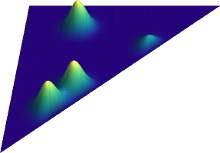# Topological Machine Learning

Topological data analysis uses topological properties of datasets as a source for data analysis. The dominant mathematical tool for this is persistent homology, where, roughly speaking, the lifespan of loops and holes of different dimensions are considered in a growing topological space.1 The times of birth and death of all such holes give rise to the so-called persistence diagram of a certain dimension, and those are often a valuable source of information.

Topological machine learning. In [Rei*14, RHBK*15] we built a bridge between topological data analysis and machine learning by constructing a so-called positive-definite kernel on the space of persistence diagrams. Once such a kernel is available, a vast body of machine learning tools is available to topological data analysis, such as support vector machines, k-means, or kernel-PCA.

Our kernel is a positive-definite multi-scale kernel and motivated by a heat diffusion process on a half-plane, where the boundary condition plays an important role to guarantee topological stability of the kernel. (Roughly speaking, points in the persistence diagram that are close to the diagonal must have less influence in the kernel.) In particular, we can show stability w.r.t. 1-Wasserstein distance and we show that non-trivial additive kernel on persistence diagrams cannot be stable w.r.t. p-Wasserstein distance where $$1 < p \le \infty$$.

So let $$D$$ denote a persistence diagram, that is, a multi-set of points in the domain $$\Omega := \{ (x, y) \in \mathbb{R}^2 \; : \; y \ge x \}$$. Roughly speaking, we place at each point on of $$D$$ a Dirac delta distribution and take the sum of those as the initial condition to heat-diffusion problem on the domain $$\Omega$$ with the boundary condition that the solution is to be zero at the boundary. For a fixed diffusion time $$\sigma > 0$$ we can assign to each diagram $$D$$ the solution to this heat-diffusion problem. Our kernel $$k_\sigma$$ is now defined by the inner produce on the space $$L_2(\Omega)$$, where the heat-diffusion solutions live in.Universal kernel. In [Kwi*15] we presented a modified version of the above kernel that is universal in the sense of Steinwart2. Let $$C(\Omega)$$ denote the space of continuous, bounded functionals on $$\Omega$$, then a universal kernel $$k$$ on $$\Omega$$ has the property that its RKHS is dense in $$C(\Omega)$$. Universal kernels are attractive in a statistical context since the mean map via such a kernel is injective, i.e., a probability distribution can be uniquely represented in the RKHS of the kernel.

1. See for instance the Getting Started page or the Demonstration page of libstick, my C++ library for comptuing persitent homology.

2. I. Steinwart. On the influence of the kernel on the consistency of support vector machines. JMLR, 2:67– 93, 2001.